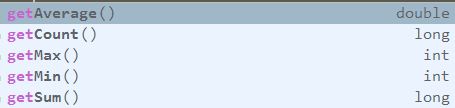• 如果您觉得本站非常有看点，那么赶紧使用Ctrl+D 收藏吧

# 一. 什么是 Stream

Stream 中文称为 “流”，通过将集合转换为这么一种叫做 “流” 的元素序列，通过声明性方式，能够对集合中的每个元素进行一系列并行或串行的流水线操作

# 二. 流操作# 三. 常用方法

### 1. filter(T -> boolean)

``````保留年龄为 20 的 person 元素
list = list.stream()
.filter(person -> person.getAge() == 20)
.collect(toList());

collect(toList()) 可以把流转换为 List 类型

### 3. sorted() / sorted((T, T) -> int)

``````根据年龄大小来比较：
list = list.stream()
.sorted((p1, p2) -> p1.getAge() - p2.getAge())
.collect(toList());``````

``````list = list.stream()
.sorted(Comparator.comparingInt(Person::getAge))
.collect(toList());``````

### 4. limit(long n)

``````list = list.stream()
.limit(2)
.collect(toList());

### 5. skip(long n)

``````list = list.stream()
.skip(2)
.collect(toList());

tips:

• 用在 limit(n) 前面时，先去除前 m 个元素再返回剩余元素的前 n 个元素
• limit(n) 用在 skip(m) 前面时，先返回前 n 个元素再在剩余的 n 个元素中去除 m 个元素
``````list = list.stream()
.limit(2)
.skip(1)
.collect(toList());

### 6. map(T -> R)

``List<String> newlist = list.stream().map(Person::getName).collect(toList());``

newlist 里面的元素为 list 中每一个 Person 对象的 name 变量

### 7. flatMap(T -> Stream)

``````List<String> list = new ArrayList<>();

list = list.stream().map(s -> s.split(" ")).flatMap(Arrays::stream).collect(toList());``````

### 8. anyMatch(T -> boolean)

``````是否存在一个 person 对象的 age 等于 20：
boolean b = list.stream().anyMatch(person -> person.getAge() == 20);``````

### 11. findAny() 和 findFirst()

• findAny()：找到其中一个元素 （使用 stream() 时找到的是第一个元素；使用 parallelStream() 并行时找到的是其中一个元素）
• findFirst()：找到第一个元素

### 12. reduce((T, T) -> T) 和 reduce(T, (T, T) -> T)

``````计算年龄总和：
int sum = list.stream().map(Person::getAge).reduce(0, (a, b) -> a + b);

int sum = list.stream().map(Person::getAge).reduce(0, Integer::sum);``````

``````计算年龄总乘积：
int sum = list.stream().map(Person::getAge).reduce(1, (a, b) -> a * b);``````

``Optional<Integer> sum = list.stream().map(Person::getAge).reduce(Integer::sum);``

### 15. forEach()

``````
### 16. unordered()

list.stream().forEach(System.out::println);``````

``````向数据库插入新元素：
list.stream().forEach(PersonMapper::insertPerson);``````

# 四. 数值流

`int sum = list.stream().map(Person::getAge).reduce(0, Integer::sum);` 计算元素总和的方法其中暗含了装箱成本，`map(Person::getAge)` 方法过后流变成了 Stream 类型，而每个 Integer 都要拆箱成一个原始类型再进行 sum 方法求和，这样大大影响了效率。

• mapToInt(T -> int) : return IntStream
• mapToDouble(T -> double) : return DoubleStream
• mapToLong(T -> long) : return LongStream
``IntStream intStream = list.stream().mapToInt(Person::getAge);``

``LongStream longStream = list.stream().mapToInt(Person::getAge);``

``Stream<Integer> stream = intStream.boxed();``

• sum()
• max()
• min()
• average() 等…

## 3. 数值范围

IntStream 与 LongStream 拥有 range 和 rangeClosed 方法用于数值范围处理

IntStream ： rangeClosed(int, int) / range(int, int) LongStream ： rangeClosed(long, long) / range(long, long)

``````求 1 到 10 的数值总和：
IntStream intStream = IntStream.rangeClosed(1, 10);
int sum = intStream.sum();``````

• rangeClosed(1, 100) ：[1, 100]
• range(1, 100) ：[1, 100)

## 4. 汇总

### （1）counting

``long l = list.stream().collect(counting());``

``long l = list.stream().count();``

### （2）summingInt ，summingLong ，summingDouble

summing，没错，也是计算总和，不过这里需要一个函数参数

``int sum = list.stream().collect(summingInt(Person::getAge));``

``int sum = list.stream().mapToInt(Person::getAge).sum();``

``int sum = list.stream().map(Person::getAge).reduce(Interger::sum).get();``

### （3）averagingInt，averagingLong，averagingDouble

``Double average = list.stream().collect(averagingInt(Person::getAge));``

``OptionalDouble average = list.stream().mapToInt(Person::getAge).average();``

### （4）summarizingInt，summarizingLong，summarizingDouble

``IntSummaryStatistics l = list.stream().collect(summarizingInt(Person::getAge));``

IntSummaryStatistics 包含了计算出来的平均值，总数，总和，最值，可以通过下面这些方法获得相应的数据## 3. 取最值

maxBy，minBy 两个方法，需要一个 Comparator 接口作为参数

``Optional<Person> optional = list.stream().collect(maxBy(comparing(Person::getAge)));``

``Optional<Person> optional = list.stream().max(comparing(Person::getAge));``

## 4. joining 连接字符串

``````String s = list.stream().map(Person::getName).collect(joining());

``````String s = list.stream().map(Person::getName).collect(joining(","));

joining 还有一个比较特别的重载方法：

``````String s = list.stream().map(Person::getName).collect(joining(" and ", "Today ", " play games."));

## 5. groupingBy 分组

groupingBy 用于将数据分组，最终返回一个 Map 类型

``Map<Integer, List<Person>> map = list.stream().collect(groupingBy(Person::getAge));``

### 多级分组

groupingBy 可以接受一个第二参数实现多级分组：

``Map<Integer, Map<T, List<Person>>> map = list.stream().collect(groupingBy(Person::getAge, groupBy(...)));``

### 按组收集数据

``Map<Integer, Integer> map = list.stream().collect(groupingBy(Person::getAge, summingInt(Person::getAge)));``

``groupingBy(Person::getAge)``

``groupingBy(Person::getAge, toList())``

## 6. partitioningBy 分区

``````根据年龄是否小于等于20来分区
Map<Boolean, List<Person>> map = list.stream()
.collect(partitioningBy(p -> p.getAge() <= 20));

{
false=[Person{name='mike', age=25}, Person{name='tom', age=30}],
true=[Person{name='jack', age=20}]
}``````

# 7. 并行

`` int i = Stream.iterate(1, a -> a + 1).limit(100).parallel().reduce(0, Integer::sum);``

ArrayList 极佳